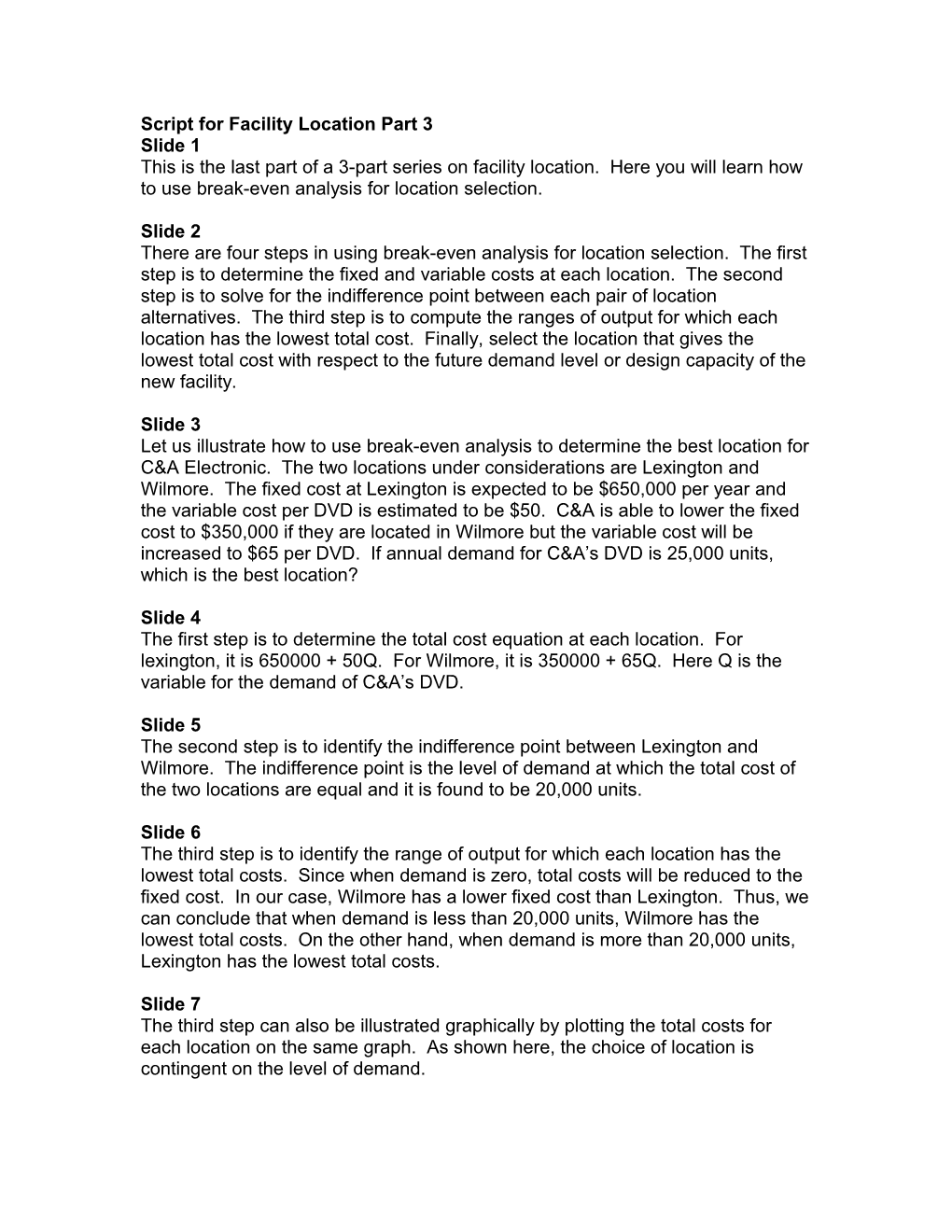This Is the Third Part of the 3-Part Series on Facility LocationScript for Facility Location Part 3

Slide 1

This is the last part of a 3-part series on facility location. Here you will learn how to use break-even analysis for location selection.

Slide 2

There are four steps in using break-even analysis for location selection. The first step is to determine the fixed and variable costs at each location. The second step is to solve for the indifference point between each pair of location alternatives. The third step is to compute the ranges of output for which each location has the lowest total cost. Finally, select the location that gives the lowest total cost with respect to the future demand level or design capacity of the new facility.

Slide 3

Let us illustrate how to use break-even analysis to determine the best location for C&A Electronic. The two locations under considerations are Lexington and Wilmore. The fixed cost at Lexington is expected to be \$650,000 per year and the variable cost per DVD is estimated to be \$50. C&A is able to lower the fixed cost to \$350,000 if they are located in Wilmore but the variable cost will be increased to \$65 per DVD. If annual demand for C&A’s DVD is 25,000 units, which is the best location?

Slide 4

The first step is to determine the total cost equation at each location. For lexington, it is 650000 + 50Q. For Wilmore, it is 350000 + 65Q. Here Q is the variable for the demand of C&A’s DVD.

Slide 5

The second step is to identify the indifference point between Lexington and Wilmore. The indifference point is the level of demand at which the total cost of the two locations are equal and it is found to be 20,000 units.

Slide 6

The third step is to identify the range of output for which each location has the lowest total costs. Since when demand is zero, total costs will be reduced to the fixed cost. In our case, Wilmore has a lower fixed cost than Lexington. Thus, we can conclude that when demand is less than 20,000 units, Wilmore has the lowest total costs. On the other hand, when demand is more than 20,000 units, Lexington has the lowest total costs.

Slide 7

The third step can also be illustrated graphically by plotting the total costs for each location on the same graph. As shown here, the choice of location is contingent on the level of demand.

Slide 8

The last step is to select the location that gives the lowest cost for the expected future demand. Since the future demand is given to be 25,000 units which is more than the indifference point of 20,000 units, Lexington should be chosen as the location for the new facility.